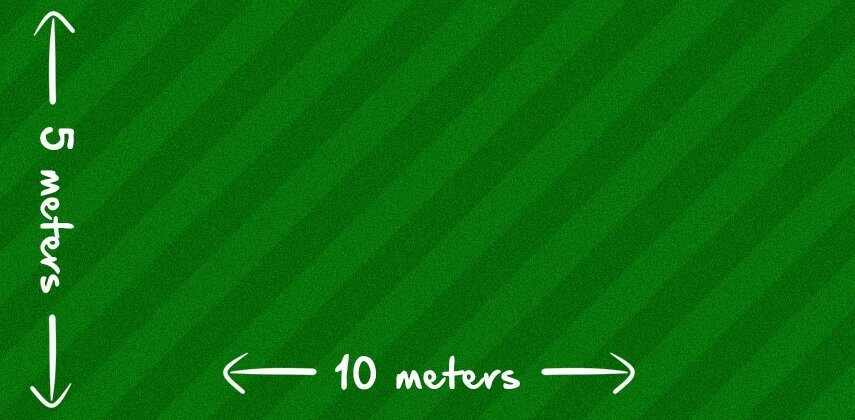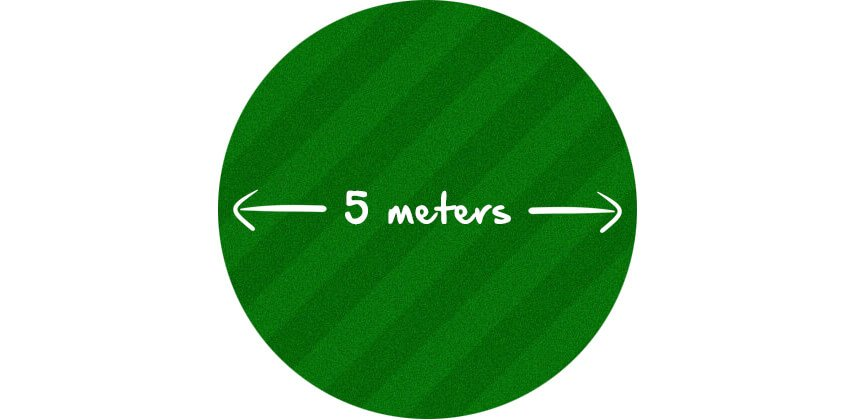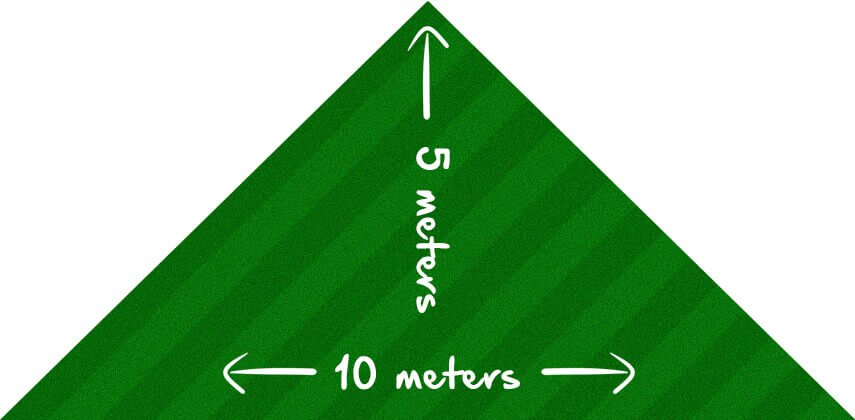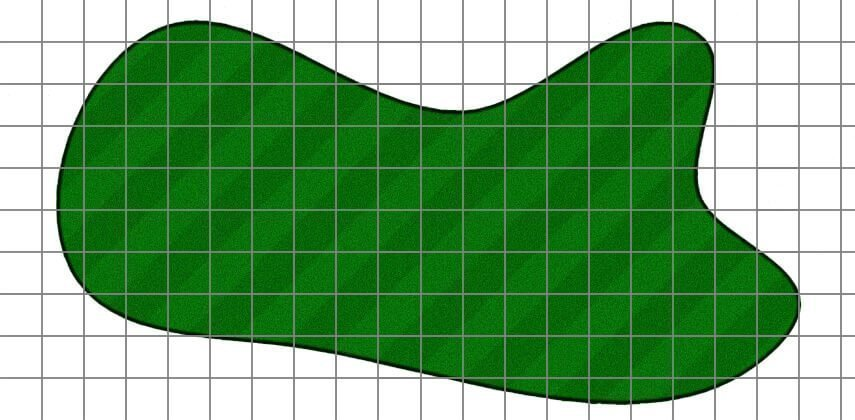# How Much Turf Do I Need? Use Our Turf Calculator to Work it Out Quickly And Easily

Contents

Use my turf calculator to figure out how many rolls of turf you need for your next project.

One roll of turf is equal to 1m².

I always recommend adding 5% to your order. There’s nothing more infuriating than being a few rolls short when laying a new lawn. This 5% will account for any wastage and shaping.

[CP_CALCULATED_FIELDS id=”7″]

## How to Calculate How Much Turf You Need

If you’d rather work it out for yourself I’ll show you exactly how in just a moment firstly…

…Use a tape measure – ALWAYS.

Don’t use your steps as a measurement and ‘guesstimate’ it. This will either end up with you buying too much turf and wasting money or, not buying enough and being short.

Secondly, rolls of turf are supplied by the square meter so take your measurements in meters. Measuring in feet or yards and then converting adds another layer of unnecessary confusion.

So now that’s covered let’s me show you how to accurately work out how much turf you need.

### How to Measure Rectangular or Square LawnsArea = length x width

Square and rectangular lawns are the easiest type measure.

Simply multiply the length of your lawn by the width.

For example, if your lawn is 10 meters long and 5 meters wide, the equation will look like this:

10 x 5 = 50

That’s 50 square meters.

As rolls of turf come in square meters, you’d need 50 rolls.

### How to Measure Circular LawnsThis is slightly more complex but still, it’s pretty simple.

Take the diameter of your lawn (that’s the measurement across the circle at its widest points) and divide it by two to get your radius. Let’s call it ‘R’.

The equation you need is R x R x 3.14.

So if your circular lawn is 5 meters across, this is how you’d work it out;

5 (diameter) / 2 = 2.5 (R)

2.5(R) x 2.5 (R) x 3.14 = 19.63

That’s 20.6 square meters, or 21 rolls of turf.

### How to Measure Triangular LawnsArea = 1/2 longest side x height

To work out how much turf you need to a triangular lawn, take the length of the longest side of the triangle and divide it by two.

Then, multiply that number by the height.

For example, if the longest side of your triangle is 10 meters and the height is 5 meters, this is how you’d work it out;

10 (longest side) / 2 = 5 x 5 (height) = 25

Or 25 square meters, or 25 rolls of turf.

## When a Turf Calculator Doesn’t Work

As helpful as a turf calculator is, it won’t help if you have odd or uneven shaped lawns.

In this case, these best way to work out how much turf you’ll need is to measure it manually.

This can be tricky to do so it’s worth ordering a little more turf to make sure you have enough. In a case like this, having too much is better than not having enough.

### How to Measure Odd Shaped LawnsFirstly, take a piece of graph paper and allocate one large square to a square meter.

Then, measure the length and width of your garden and mark it out on your paper.

Once you have done that, measure any permanent features like patios, walls, garden buildings et.c and draw them onto your plan. This will help you draw your lawn to a better scale.

Now measure the distance of the edges of your lawn from those permanent features and mark them on your plan before drawing the overall outline of the lawn.

Once you have done this, all that’s left to do is to count the full squares, then take an educated guess of the square meterage of the partial squares and add the two numbers together.

This will give you a fairly accurate figure.

## In Conclusion

I hope you find my turf calculator useful and I hope it makes working out how much turf you need a bit easier.

And if you prefer to measure things manually, I hope my formulas help.

If you have any questions or comments regarding working out how much turf you need for your next project, drop me a comment below and I’ll get back to you ASAP.